## Capturas de pantalla

•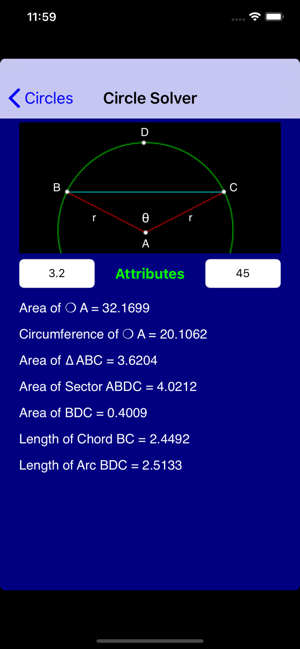•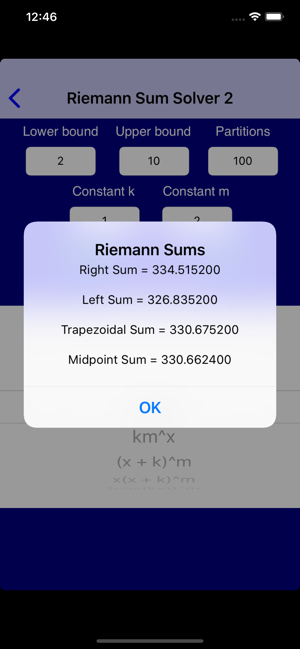•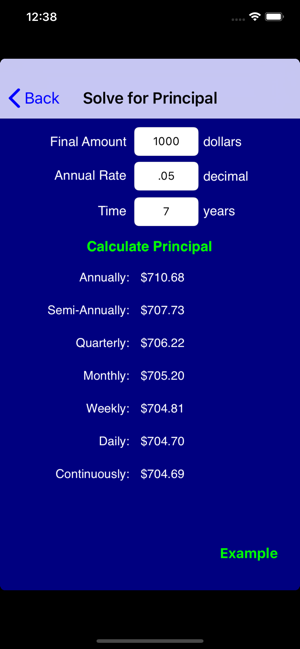•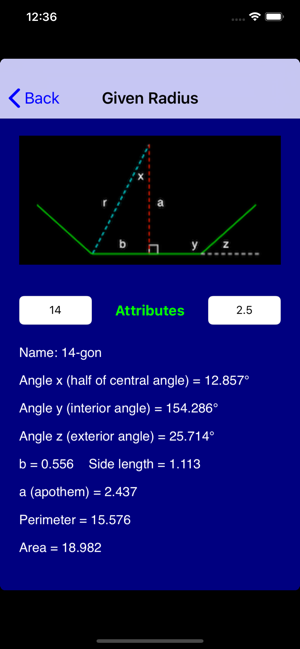•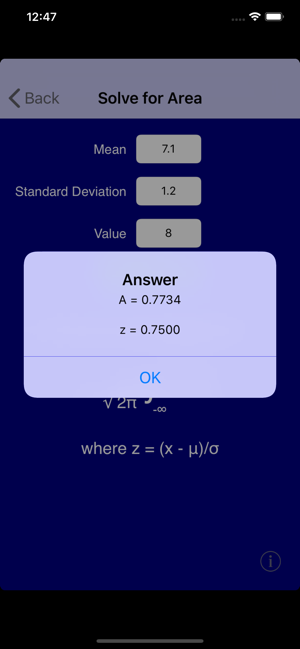•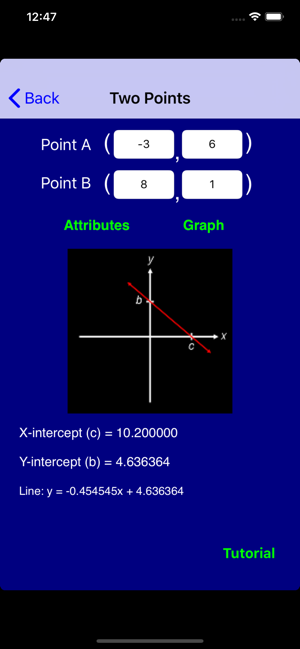•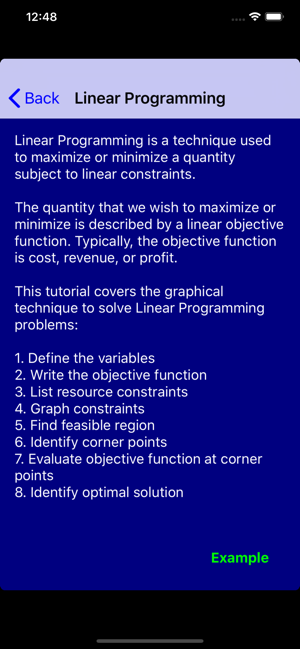•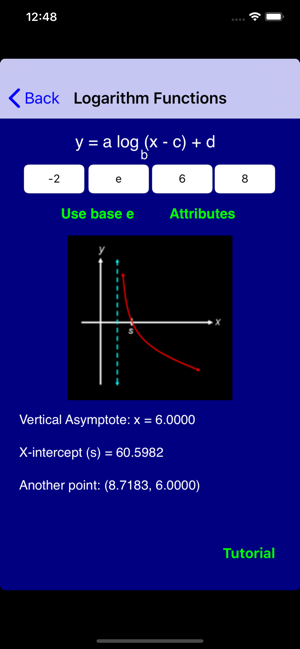•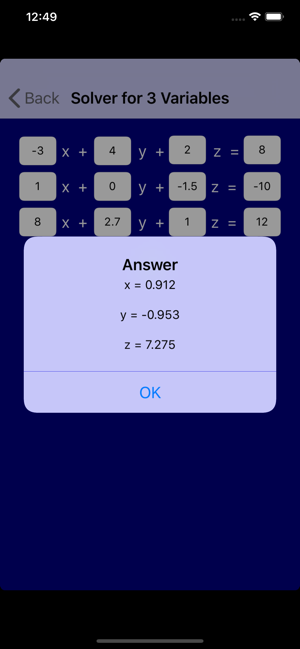•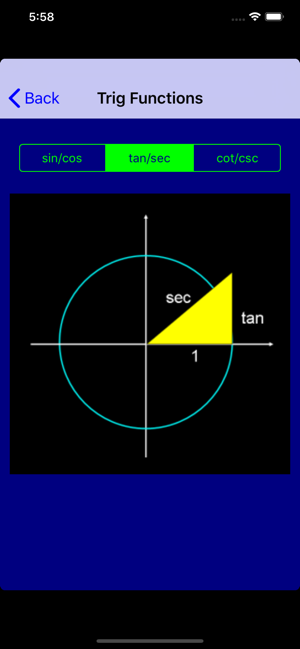## Descripción

Get six applications for the price of two and ace your math classes.

Rated 5/5 by AppleBitesize: www.applebitesize.com/posts/math-pro-review

"Math Pro" will take you through high-school Math and beyond. It is a powerful tool that is overflowing with the tutorials, examples, and solvers from the following applications: Algebra Pro, Geometry Pro, Probability Pro, Statistics Pro, PreCalculus Pro, and Calculus Pro.

"Math Pro" is completely searchable, and it covers an astonishing array of topics:

- Classifying Numbers
- Points and Lines
- Systems of Equations
- Word Problems
- Inequalities
- Linear Programming
- Direct and Inverse Variation
- Polynomials
- Zeros and End Behavior
- Synthetic Division
- Remainder Theorem
- Factoring
- Domains and Ranges
- Compositions of Functions
- Function Transformations
- Inverse Functions
- Piecewise Functions
- Perimeter, Area and Volume
- Angles
- Segments
- Midpoints
- Distance Formula
- Distance from Point to Line
- Law of Sines
- Law of Cosines
- Solving Triangles
- Similar Triangles
- Congruent Triangles
- 30-60-90 Triangles
- 45-45-90 Triangles
- Medians
- Altitudes
- Perpendicular Bisectors
- Orthocenters
- Circumcenters
- Centroids
- Circles
- Regular Polygons
- Logic Statements
- Postulates and Theorems
- Geometric Proofs
- Venn Diagrams
- Bayes' Theorem
- Survey Results
- Odds
- Permutations
- Combinations
- Taking Balls from Urns
- Replacement vs. Non-Replacement
- Independent Events
- Dice
- Roulette
- Lottery
- Poker
- General Card Problems
- Expected Value
- Birthday Problem
- Five Number Summaries
- Outliers
- Linear Regression
- Chebyshev's Theorem
- Normal Distribution
- Binomial Distribution
- Poisson Distribution
- Exponential Distribution
- Chi Squared Distribution
- Central Limit Theorem
- T-Tests
- Confidence intervals
- Uniform Random Number Generator
- Binomial Random Number Generator
- Gaussian Random Number Generator
- Poisson Random Number Generator
- Exponential Random Number Generator
- Logarithms
- Logarithmic Functions
- Exponential Functions
- Rational Functions
- Compound Interest
- Graph Symmetry
- Conic Sections
- Arithmetic Sequences
- Geometric Sequences
- Matrices
- Binomial Expansion
- Complex Numbers
- Rectangular and Polar Notation
- Standard Unit Circle
- Unit Circle Representations of the Six Trig Functions
- Attributes and Graphical Representations of the Six Trig Functions
- Changes in Amplitude, Period, Horizontal Shifts and Vertical Shifts
- Formulas and Identities
- Inverse Trig Functions
- Reference Angles
- Simplifying Trig Expressions
- Angles of Elevation and Depression
- Polar curves: Limacons, Rose Curves, and Lemniscates
- Limits
- Continuity
- Differentiability
- RDQ, LDQ, SDQ and NDQ
- Equations of Tangent Lines
- Partial Derivatives
- Implicit Differentiation
- Chain Rule
- Product Rule
- Quotient Rule
- Related Rates Problems
- Optimization Examples (Min/Max)
- Derivatives of Inverse Functions
- Taylor and Maclaurin Series
- Derivative Tables
- Derivatives of Integrals
- Arc Length
- Riemann Sums
- Fluid Problems
- Double Integrals
- Integration by Parts
- Definite and Indefinite Integrals
- Volumes of Solids of Revolution
- Cross Sectional Volumes
- Integral tables
- Position, Velocity and Acceleration
- Calculus Theorems

I welcome all comments, questions, and suggestions: lfeldman65@gmail.com

Versión 7.8

- Corrected bug in Riemann Sum Solver 2

## Valoraciones y reseñas

1.0 de 5
1 valoración

1 valoración

Iuser1014 ,

### No soporta decimales

En los dialogos en los que hay que introducir cantidades numéricas con decimales (por ej., probabilidades) se muestra el teclado numérico...españól, donde el punto decimal americano es una coma y da error. Imposible workaround. Hasta que no lo solucionen, app inútil.

## Información

Proveedor
Larry Feldman
Tamaño
12.7 MB
Categoría
Educación

Requiere iOS 9.0 o posterior. Compatible con iPhone, iPad y iPod touch.

Idiomas

Inglés

17+
Acceso web no restringido
Precio
2,29 €

•